Mathematical and Physical Journal
for High Schools
Issued by the MATFUND Foundation
 Already signed up? New to KöMaL?

# KöMaL Problems in Physics, November 2013

Show/hide problems of signs:## Problems with sign 'M'

Deadline expired on December 10, 2013.

M. 336. Mount an axle to the cap of a half-litre PET-bottle, and measure the period of the swinging bottle about this axle. Vary the amount of liquid in the bottle and determine the amount of liquid in the bottle for which the period is the greatest.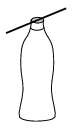(6 pont)

statistics## Problems with sign 'P'

Deadline expired on December 10, 2013.

P. 4572. A vehicle covers equal distances at speeds of 1 m/s, 2 m/s, 3 m/s, 4 m/s and 5 m/s. What is its average speed calculated for the total distance covered?

(3 pont)

solution (in Hungarian), statistics

P. 4573. The coefficient of static friction between the fixed inclined plane of angle of inclinationand the block of mass M, shown in the figure, is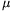. The radii of the two alike pulleys are r and R (R>r), there is no friction at the axles, and the mass of the pulleys is negligibly small with respect to the other masses. Two pieces of rope are vertical, and one is parallel to the slope. The mass of the block at the bottom is m. At what m/M mass-ratio can the system be in equilibrium?

Data:=30o,=0,2 and.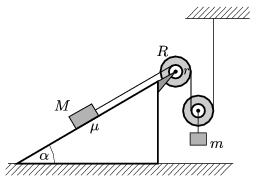(4 pont)

solution (in Hungarian), statistics

P. 4574. A right triangle shaped wedge of mass m=0.5 kg and of angle 30o is placed to the top of a fixed slope of length=1.8 m, and of angle of elevation=30o, as shown in the figure. The vertical height of the wedge is exactly half of the height of the slope H. The wedge is loaded with the small block of mass m as shown in the figure.

This system is assembled in two samples. In case of one of them the small block is fixed to the wedge and in the other case the block is not fixed. The two wedges are released from rest at the same time. Friction is negligible at any surfaces.

a) Determine the ratio of the times during which the wedges reach the bottom of the slopes.

b) What are the forces exerted by the small blocks on the wedges in the two cases?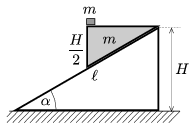(5 pont)

solution (in Hungarian), statistics

P. 4575. According to Julie some space probes are accelerated by the so called swing-by (or gravitational slingshot) effect, and to use the gravity assistance the space probe just has to pass a planet appropriately. According to Joe the space probe accelerates only when it approaches the planet and it slows down to its original speed while it moves away from the planet, because in the gravitational field of the planet the potential energy depends on only the distance measured from the centre of mass of the planet. So the effect only increases the average speed of the probe, but this is also advantageous. Who is right?

(4 pont)

solution (in Hungarian), statistics

P. 4576. The outer radius of a spherical shell, made of insulating material, is r2=0.2 m, and its inner radius is r1=0.15 m. The relative dielectric constant of its material is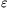r=8.4. The shell is charged positively, and the charge is distributed evenly such that the charge density is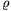=10-5 C/m3. Measured from the centre of the spherical shell where are those points at which the magnitude of the electric field strength is

aE1=3.103 V/m,

bE2=3.104 V/m?

(5 pont)

solution (in Hungarian), statistics

P. 4577. What is the equivalent resistance of the infinite resistor chain, shown in the figure, between the points A and B? Plot the equivalent resistance versus q graph.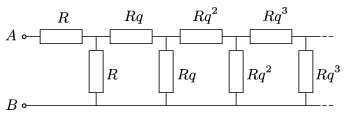(5 pont)

solution (in Hungarian), statistics

P. 4578. There is a glowing filament 30 cm from a 5-dioptre converging lens, whilst on the other side of the lens, on the same principal axis, 50 cm away from the lens there is a -2 dioptre diverging lens. Where should the screen be placed if we would like to find the image of the filament? What is the size of the image of the 2-cm long filament?

(4 pont)

solution (in Hungarian), statistics

P. 4579. Traces of Argon were found in one of the most ancient stone of the Earth. The stone was powdered in vacuum, and it was found that the number of Argon atoms is 1/10000 of the number of Potassium atoms. Theatoms are radioactive and their half-life is 1.18.109 years. 11% of the daughter elements areatoms which are stable. Now 0.0118% of all the potassium atoms isisotopes. How old is the stone?

(5 pont)

solution (in Hungarian), statistics

P. 4580. A thin, light, elastic rod sticks out of the wall of an old house. In which case will its end be bent more, if a pigeon sits to its middle or if a blackbird of mass one-quarterth of the mass of the pigeon sits to the end of the rod?

(5 pont)

solution (in Hungarian), statistics

P. 4581. A frictionlessly moveable piston of mass m confines n=0.25 moles of diatomic gas at a temperature of T1=300 K in a vertical cylinder, closed at its bottom, of cross section A=2 dm2. The piston is at a distance of h1=3 dm from the bottom and the ambient pressure is p0=105 Pa.

The gas is heated in two steps. The first step lasts until the piston reaches the stoppers, in this stage the piston is h2=5 dm from the bottom of the cylinder. The added heat in the second step, which begins when the piston reaches the stopper, istimes as much as the added heat in the first step.

a) Determine the mass m of the piston.

b) What is the total force exerted by the stoppers on the piston at the end of the second heating step?

c) Plot the pressure of the gas as a function of the absolute temperature.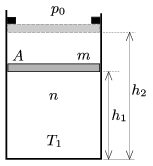(5 pont)

solution (in Hungarian), statistics

P. 4582. An astronaut in the International Space-station attaches himself to the four vertices of a regular tetrahedron shaped frame with 4 springs. The mass of the springs and their rest length are negligible, their spring constants are D1=150 N/m, D2=250 N/m, D3=300 N/m and D4=400 N/m. - For the sake of simplicity - the astronaut is considered pointlike, its mass is m=70 kg. What is the period of the oscillation of the astronaut if he is displaced from his equilibrium position and released?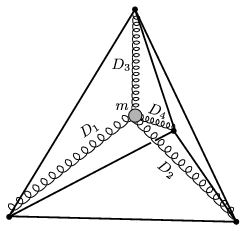(6 pont)

solution (in Hungarian), statistics